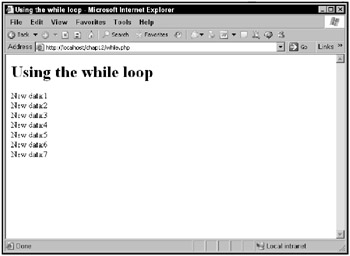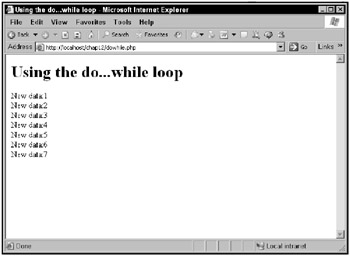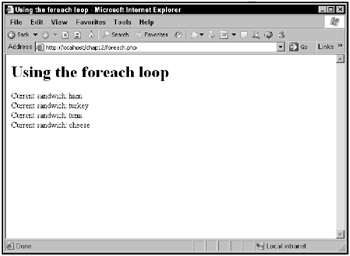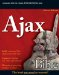Looping with the while loop

The while loop keeps executing its code while an expression remains true. Here’s what this loop looks like:

while (expression)    statement

In this case, statement can be a compound statement, surrounded in curly braces. This loop keeps going as long as the condition expression remains true.

Here’s an example, while.php. This example displays the value in a variable and increments it until it becomes greater than 8.

The while loop is set up like this:

<html>    <head>        <title>            Using the while loop        </title>    </head>        <body>        <h1>            Using the while loop        </h1>        <?            \$data = 1;              while (\$data < 8){            .            .            .            }        ?>     </body> </html>

then you can display the current value of the variable and increment that value like this:

<html>     <head>         <title>             Using the while loop         </title>     </head>     <body>         <h1>             Using the while loop         </h1>         <?             \$data = 1;             while (\$data < 8){                 echo "New data:", \$data, "<br>";                 \$data += 1;             }         ?>     </body> </html>

The results are shown in Figure 12.14, where the loop terminates when the value in the variable reaches 8.Figure 12.14: Using the while loop

Looping with the do...while loop

There’s another version of the while loop: the do...while loop, which checks its condition at the end of the loop, not the beginning. That means the loop’s statement is always executed at least once (which is good if that statement sets the condition you want to test to see whether the loop should keep looping):

do     statement while (expression)

Here’s what the previous while loop example would look like as a do...while loop instead, dowhile.php:

<html>     <head>         <title>             Using the do...while loop         </title>     </head>     <body>         <h1>             Using the do...while loop         </h1>         <?            \$data = 1;            do {                echo "New data:", \$data, "<br>";                \$data += 1;            } while (\$data < 8)         ?>     </body> </html>

You can see the results in Figure 12.15.Figure 12.15: Using the do...while loop

There’s one more loop in PHP as well, and it’s an important one: the foreach loop, which you use with collections of data items like arrays.

Looping with the foreach loop

The PHP foreach loop is designed to be used with collections of data items, like arrays. Here’s one way to use this loop:

foreach (array as \$value)   statement

In this case, array is an array, and \$value a variable. Each time through the loop, \$value holds the next element from the array.

Here’s an example, foreach.php. In this example, you can create an array that the foreach loop will loop over, and set up the foreach loop like this:

<html>     <head>       <title>Using the foreach loop</title>   </head>     <body>         <h1>Using the foreach loop</h1>             <?                 \$array = array("ham", "turkey", "tuna",                   "cheese");                 foreach (\$array as \$sandwich) {                 .                 .                 .                 }             ?>     </body> </html>

Now each time through the loop, \$sandwich will hold the next successive element from \$array, so you can display the current sandwich like this:

<html>     <head>       <title>Using the foreach loop</title>   </head>     <body>         <h1>Using the foreach loop</h1>             <?                 \$array = array("ham", "turkey", "tuna",                   "cheese");                 foreach (\$array as \$sandwich) {                    echo "Current sandwich: \$sandwich <br>";                 }             ?>     </body> </html>

That’s all you need. The results are shown in Figure 12.16. As you can see, the foreach loop was able to loop over all the items in the array automatically.Figure 12.16: Using the foreach loop

The foreach loop is a good one to use with collections like arrays because you don’t have to worry about making sure you set up the loop index in a for loop just right, so the loop terminates after you’ve looped over every item in the collection.Ajax Bible
ISBN: 0470102632
EAN: 2147483647
Year: 2004
Pages: 169

Similar book on Amazon